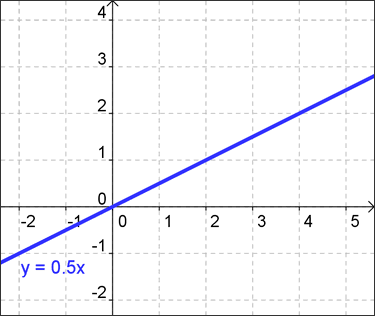# Direct Variation

When two variables are related in such a way that the ratio of their values always remains the same, the two variables are said to be in direct variation.

In simpler terms, that means if A is always twice as much as B, then they directly vary. If a gallon of milk costs $3, and I buy 1 gallon, the total cost is$3. If I buy 10 gallons, the price is \$30. In this example the total cost of milk and the number of gallons purchased are subject to direct variation -- the ratio of the cost to the number of gallons is always 3.

To be more "geometrical" about it, if y varies directly as x, then the graph of all points that describe this relationship is a line going through the origin (0, 0) whose slope is called the constant of variation. That's because each of the variables is a constant multiple of the other, like in the graph shown below:## Key concepts of direct variation:

#### How do I Recognize Direct Variation in an Equation?

The equation $$\frac{y}{x} = 6$$ states that y varies directly as x, since the ratio of y to x (also written y:x) never changes. The number 6 in the equation $$\frac{y}{x} = 6$$ is called the constant of variation. The equation $$\frac{y}{x} = 6$$ can also be written in the equivalent form, $$y = 6x$$. That form shows you that y is always 6 times as much as x.

Similarly, for the equation $$y=\frac{x}{3}$$, the constant of variation is $$\frac{1}{3}$$. The equation tells us that for any x value, y will always be 1/3 as much.

#### Algebraic Interpretation of Direct Variation

For an equation of the form $$y = kx$$, multiplying x by some fixed amount also multiplies y by the SAME FIXED AMOUNT. If we double x, then we also double the corresponding y value. What does this mean? For example, since the perimeter P of a square varies directly as the length of one side of a square, we can say that P = 4s, where the number 4 represents the four sides of a square and s represents the length of one side. That equation tells us that the perimeter is always four times the length of a single side (makes sense, right?), but it also tells us that doubling the length of the sides doubles the perimeter (which will still be four times larger in total).

#### Geometric Interpretation of Direct Variation

The equation $$y = kx$$ is a special case of linear equation ($$y=mx+b$$) where the y-intercept equals 0. (Note: the equation $$y = mx + b$$ is the slope-intercept form where m is the slope and b is the y-intercept). Anyway, a straight line through the origin (0,0) always represents a direct variation between y and x. The slope of this line is the constant of variation. In other words, in the equation $$y = mx$$, m is the constant of variation.

## Example A:

If y varies directly as x, and $$y = 8$$ when $$x = 12$$, find k and write an equation that expresses this variation.

#### Plan of Attack:

Plug the given values into the equation $$y = kx$$.

Solve for k.

Then replace k with its value in the equation $$y = kx$$.

#### Step-by-Step:

Start with our standard equation: $$y = kx$$

Insert our known values: $$8 = k*12$$

Divide both sides by 12 to find k: $$\frac{8}{12} = k$$

$$\frac{2}{3} = k$$

Next: Go back to $$y = kx$$ and replace k with $$\frac{2}{3}$$.

#### Result:

$$y = \frac{2}{3}*x$$

## Example B:

If y varies directly as x, and $$y = 24$$ when $$x = 16$$, find y when $$x = 12$$.

#### Plan of Attack:

When two quantities vary directly, their ratio is always the same. We'll create two ratios, set them equal to each other, and then solve for the missing quantity.

#### Step-by-Step:

The given numbers form one ratio which we can write as $$\frac{y}{x}$$: $$\frac{24}{16}$$

To find y when $$x=12$$ we setup another ratio: $$\frac{y}{12}$$

#### Solve:

By definition, both ratios are equal:

$$\frac{24}{16} = \frac{y}{12}$$

Multiply each side by 12 to solve for y:

$$\frac{24}{16}*12 = y$$ $$y = \frac{3}{2}*12$$

#### Result:

y = 18 when x = 12

Got a basic understanding of direct variation now? If you still need more help, try searching our website (at the top of the page) for a more specific question, or browse our other algebra lessons. Sometimes it helps to have a subject explained by somebody else (a fresh perspective!) so you may also be interested in another lesson on direct variation, such as this page that provides examples solving direct variation.